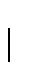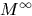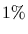Next: Dayhoff Matrices Up: Modeling Evolution Previous: Modeling Evolution

## Point Accepted Mutations

The definition of matrix M describes mutation over a given period of evolution. In order to procede, we must quantify this change in a mathematically meaningful way. Dayhoff et. al. introduced the term PAM (point accepted mutation) unit. A 1-PAM unit is the amount of evolution which will change, on average,of the amino acids. In mathematical terms, this is expressed as a matrix M such thatwhere fi is the frequency of the ith amino acid. (Recall that Mii represents the probability amino acid i does not change.)

If we have a probability or frequency vector p, the productgives the probability vector (or the expected frequency of p) after an evolution equivalent to 1-PAM unit.

Alternatively, if we start with amino acid i (a probability vector which contains a 1 in position i and 0s in all others),(the ith column of M) is the corresponding probability vector after one unit of random evolution.

After k units of evolution (a k-PAM evolution), a frequency vector p will be changed into the frequency vector.

Note that PAM distance does not correlate in any immediate way to chronological time. Evolutionary rates may be very different between species and proteins.Next: Dayhoff Matrices Up: Modeling Evolution Previous: Modeling Evolution
Gaston Gonnet
1998-09-15